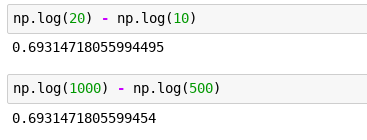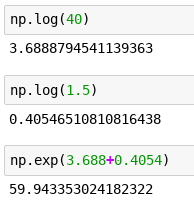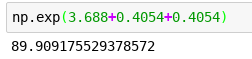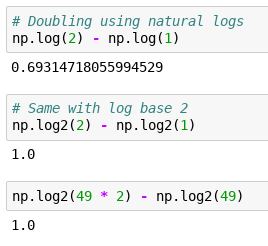That’s helpful feedback. Perhaps as a community we can try to explain some of the terms and concepts here, and then turn them into a web page? Could you let us know some of the terms or concepts you found tricky to understand?

Great lesson. Again. And yes, I also need a better brain machine for parts of this one (+ lots of Python skill)@jeremy, something useful that I thought I had commented but I see now I had not: My prefered tool for random forests is… Xgboost. (Yes, I do mean random forests).Performance-wise it is probably the best implementation of random forests, in R at least.

To proxy a random forest with Xgboost one must use number of parallel trees > 1, plus some other minor tweaks. Only difference, it will not bootstrap rows but sample them. But can handle quite big datasets, and probably, (I haven’t used tree interpreter in rf mode yet, only boosting mode) opens the door to using the tree interpreter. And, anyway… very nice performing RF tool!

3 Likes

I suspect that this might not be easy to explain - if so, no worries and sorry to bother you- but would there be an easy way to go conceptually from random forest to gradient boosting? I tried researching xgboost but never got far and none of what I read really stuck with me nor made a lot of senseBut than I had the same experience with random forrests and I think I get them@radek, Boosting is just another way of aggregating, usually trees (but could be other models).

Maybe good way to visualize it: A forest is “horizontal”. Boosting is “vertical”, you grow a tree, then you grow another one that will improve the residuals of the first one… Boosting is models correcting their mistakes sequentially. Don’t know if that makes you closer to intuition about it…

2 Likes

It does help - thank you1 Like

I have been thinking about this a bit and also some time ago in BetterExplained books I read this interesting take on exponents being likened to microwave ovens which led me to this…If we have cars and would like to know what fitting an AC will do to its price in absolute terms - how many dollars more it will be worth - we would just use prices in dollars.

But then it is as if we were saying that whether we stick an AC in a jaguar or in a Fiat 126p(Tom Hanks seems to have a thing for them though do not know the whole story)

we would want to be able to predict the difference in value - here 1000\$ more and here 1000\$ doesn’t make sense (you can buy 1/60 of a jag for 1000\$ and probably like 3 or 4 fiats 126p)

So we ask a different question - with respect to the base price, how much will fitting an AC increase it? 0.1 on the logarithmic scale will ‘grow’ both values by the same amount relative to their starting magnitude!

Maybe this has some more significance that I fail to notice yet and also apologies if this was explained in a lecture already but I am a bit behind, even more so on the notebooks than watching the lectures.

This is crazy:
Here, have 40\$, can you grow it for me by half?ah wait… but I’d like you to grow it by half… and then by half again… (this mimics getting an AC and then adding a set of rims onto the cars / bulldozers which prices we are are predicting)0.4054 always adds a half!!! OMG I can’t believe I didn’t know that.

BTW this logarithmic thing is witchcraft.

8 Likes

They are witches’ secret sauce.

3 Likes

Sure, well at least I think it has to do with my understanding of the maths. You know in lesson when you told “you really need to know how log and exp are working and what are their useful properties”. I mean, I understand what they do but I still don’t get why we would use them, in what situation. Basically I don’t have the intuition even if I learn about them. Same thing goes when you were giving these examples with the standard deviation, the stderr, the t-distribution, or why the number 22 etc…

I’m someone who learn my “mimic” or by finding patterns so I try to look for a case where a given concept make sense and in what context it does not. Then I try to find the relationship between what worked and what didn’t.

If someone tell me: “Hey here you can transform the dependent value with `np.log1p()` because that’s what we will use in the loss function so we better just do this now”. Well I won’t learn anything from that, I still don’t know why we do that, why we would want to use `log1p` now instead of transforming the variable at the very end when you fit it to the loss function etc… So I’ll just say: “Ok lets assume the guy is right” and then another concept come on top of that one and now I get lost…

It’s probably just me and maybe the course on linear algebra from Rachel will help me a lot with that. Maybe I should spend more time understanding the “basics” concepts of LA before going further into the course.

But as you asked me for a constructive feedback I hope it helped you understand my context and maybe, maybe, I’m not the only one in that situation (I hope so).

(I’m just reading @radek post on logarithms it seems to be what I was looking for haha)

You can totally skip that bit - I mention in the lesson that it’s just a little faster than training the model 5 times and seeing how much the validation set varies. Also, this section of the class is just a little deeper dive into the question of validation set construction, and isn’t necessary to understand for anything else. So I’d say just ignore it for now!Is there anything else in the lesson you didn’t follow?

Is there anything special about using base e for taking the log of values? If I understand it correctly, we could be taking logarithms of any base just as well, it is just that their scale (for lack of a better word) would be different? Meaning, to grow something by 50%, we might need to add differently sized chunks depending on the log base?`
The Euler number e or Logex is frequently used in mathematics because solutions of mathematical problems comes out naturally either as ef(x) or logef(x). The use of base 2 or base 10 in these cases will produce clumsy expressions.
Examples

(1) Integral of 1/cos(x) = loge(secx + tanx).

(2) Integral of 1/(1-x2) = (1/2)Loge[(1+x)/(1-x)]

(3) Integral of 1/(1+x2)1/2 = Sinh-1x = loge[x+ sqrt(1+x2)]

(4) The solution of the LODE y’’ -(a+b)y’ + aby = 0 is y =Aeax + Bebx .

(5) The expression for ex is quite neat ex = 1 +x + x2/2! + x3/3! + …xn/n! +…

(6) The expression for loge(1+x) is also neat and memorizable. Loge(1+x) = x -x2/2 + x3/3+ …

(7) The function eax is easily integrated to obtain the neat expression eax/a.and differentiated to get aeax.

(8) What do you say about Euler’s celebrated formula eiπ + 1 = 0. Quite incredible! The sum of a complex quantity and an integer equals zero

(9) eiθ = cosθ + isinθ. The examples are innumerable. When base e is used in mathematics the answers come out naturally. That is why is referred to as the natural logarithm. Using base 2 or 10 requires additional coefficients
`

2 Likes

To expand upon point (7) in the detailed answer by @ecdrid, the function \$e^x\$ when differentiated gives you the exact same function back!!
Optimization in general involves derivatives all around and using \$e\$ to take exponents (or logarithms which is the inverse operation) simplifies this and reduces the number of scaling coefficients you need to keep track of.

1 Like

Thank you for the repliesWhat I would like to know is whether there is anything special about natural logs that makes us pick them for this one particular scenario - when we take a log of a continuous variable we are trying to predict (given that we do this as we are interested in ratios and not absolute differences in magnitudes).

My guess is that there is no difference what base for the log we pick. Any other base for the log would be just as good for expressing the ratios. But asking as I am not sure and would like to confirm that I am on the right track here.

Actually it’s the constants which we need to worry about …

bit kind of Manual work or do unnecessary divides…

Apologies, I didn’t quite get you the first time!

You;re absolutely correct in that the choice of base you use to take logarithms makes no difference. Logarithms follow a very convenient propertynp.log(x)/np.log(2) = np.log2(x)

Exactly! The relation above means ratio of the chunk sizes will always be the same number for a given pair of bases.

2 Likes

Yep I get the whole idea but even if people tell me “skip that part and assume X” I just can’t. If I don’t know how it works under the hoods I just can’t resign to do it. But yeah I got the whole idea of the lesson don’t worryIs there anything else in the lesson you didn’t follow?

Thanks for asking. I was able to understand the “big picture” of the lesson but I still want to know the little details I wasn’t able to catch as I said in my previous post. But @radek explanations helped me a lothttps://www.ibm.com/developerworks/community/blogs/jfp/entry/2nd_Prize_WInning_Solution_to_Web_Traffic_Forecasting_competition_on_Kaggle?lang=en

How can we combine deep learning and Random Forest?

2 Likes

@jeremy on

When you are talking about feature interpretation.
The section where you talk about NaN and categorical variables seems clear to me.

What about NaN and continuous values lie a temperature, preasure, distance?
Like a real missing value

+1 I’ve always wanted to know this…or DL combined with Gradient Boosting (i.e. XGBoost)

Isn’t it about combining the results from different models? The models could be anything; Logistic, KNN, Naive Bayes, XGBoost, DL etc.

A rough rubric:

1. Use different models on the same training dataset and generate predictions on test dataset.
2. If you trained 5 models in step 1, you’ll now have 5 prediction arrays, for test dataset, from each model
3. Now, a simple way of mixing them is take a
(i) hard voting - look for the majority label for each record of 5 predictions - equiweighted averaging
(ii) soft voting - because a couple of those 5 models were better than the other 3, give them higher weights - weighted averaging
(iii) stacking
4. Generate final prediction for each record in test.

A much more detailed description with visual summaries is very well described in this notebook.

2 Likes